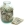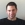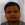### Jun 2015 May 2015 ArticlePosted 13 June in #Binary Options #Profit #Strategy Conditions
10/76
Ranking

INTRODUCTION

The binary options trading becomes more and more popular last time. Now you can try to trade binary options in the DukasCopy. But can you use your FX pairs strategy for the binary trading? Let’s see what minimum requirements are necessary for the profitable trading.
The advantage of the binary options trading is that we get fixed profit if we guess the direction of the price change. So, our strategy purpose is to guess the direction of the price change.
MODELLING OF THE PROFIT
Let’s imagine that we have the strategy with 50% probability of winning. The parameters of such strategy trading are:
• Win: 50.0%
• CAR/MDD: 0.0
• Net profit: 0.0\$
• Profit Factor: 1.0
But binary options trading has its own feature that if we guess the direction we have only a fixed percent of our bet. That’s why we will lose our money with this strategy.
So, if we want to get the profit from the binary options trading, what probability of winning do we need?
I did several simulations with the help of simple python script where each simulation has 100 trades. The parameters of the simulation were:
• Winning probability – 50%
Translate to English Show originalFecha 15 June

Of course, You are completely right! But You can use Your Forex trading strategies for the binary options trading. All You need is to use time-stop for Your orders. If You know the probability of win for the orders with fixed lilfetime, You can use it in binary options trading.driven 15 June

Remember you also need to beat the spread, so if you bet randomly you can expect a winning percentage of less than 50%.I write a small add on to your understanding... the reasoning is quite well written... but incorporating is very difficult.. hence my approach would be... towards the important schedules in the eventuality of giving sequences.. i mean towards the probability theories your income should be through which i can succeed.. i make from theoretical approaches you make less importance but the for another formula there is every success..Well I started to like the binary options on stocks, even though didn't do too well in the contest:)12/76
Ranking
INTRODUCTION
One of the most impotent conditions of successful trading is following the rules of the trading system. The main part of any trading system is trading signals, and the most common way to get the trading signals is to use the indicators.
The main purpose of the main part of indicators is to predict the price change. But can we use unusual instruments to solve this problem? Let’s try to predict the price change with the help of statistical analysis.
REGRESSION MODEL GROUNDING
So, if we want to predict the price change we need to take the initial data. The main information about the FX market is the bars. Let’s try to work only with the last N bars, more precisely only with the “Close” prices of the bars.
One of the ways to describe the global regularity of some parameter changing depending on one specific variable is the regression model (fig. 1).
Figure 1. Regression model (from Wikipedia)
We have the “Close” parameter which is depends on time of the bar. So we need to build the regression model to get the correlation between the time and the price.
According to the difficulty of the calculations and to the reasonable necessity we must decide: which model do we need? …